Spectral spread for audio signals and auditory spectrograms

Since R2019a

## Syntax

``spread = spectralSpread(x,f)``
``spread = spectralSpread(x,f,Name=Value)``
``[spread,centroid] = spectralSpread(___)``
``spectralSpread(___)``

## Description

example

````spread = spectralSpread(x,f)` returns the spectral spread of the signal, `x`, over time. How the function interprets `x` depends on the shape of `f`.```

example

````spread = spectralSpread(x,f,Name=Value)` specifies options using one or more name-value arguments.```
````[spread,centroid] = spectralSpread(___)` returns the spectral centroid. You can specify an input combination from any of the previous syntaxes.```

example

````spectralSpread(___)` with no output arguments plots the spectral spread. If the input is in the time domain, the spectral spread is plotted against time.If the input is in the frequency domain, the spectral spread is plotted against frame number. ```

## Examples

collapse all

```[audioIn,fs] = audioread("Counting-16-44p1-mono-15secs.wav"); spread = spectralSpread(audioIn,fs);```

Plot the spectral spread against time.

`spectralSpread(audioIn,fs)`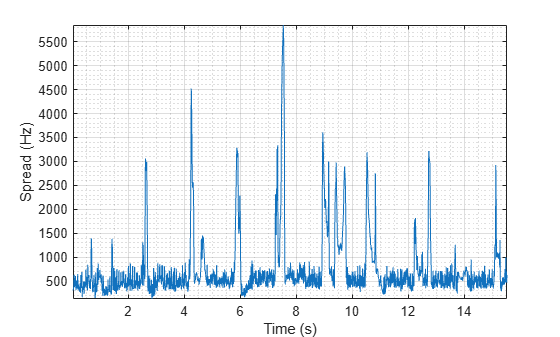Read in an audio file and then calculate the mel spectrogram using the `melSpectrogram` function. Calculate the spread of the mel spectrums over time.

```[audioIn,fs] = audioread("Counting-16-44p1-mono-15secs.wav"); [s,cf,t] = melSpectrogram(audioIn,fs); spread = spectralSpread(s,cf);```

Plot spectral spread against the frame number.

`spectralSpread(s,cf)`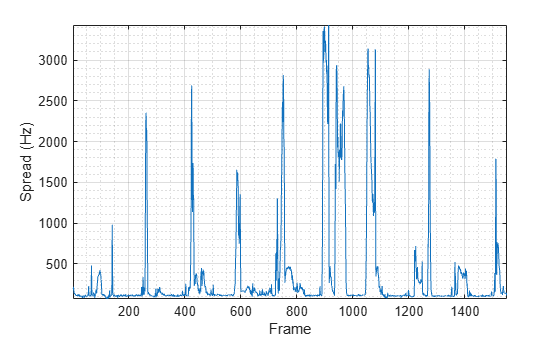`[audioIn,fs] = audioread("Counting-16-44p1-mono-15secs.wav");`

Calculate the spread of the power spectrum over time. Calculate the spread for 50 ms Hamming windows of data with 25 ms overlap. Use the range from 62.5 Hz to `fs`/2 for the spread calculation.

```spread = spectralSpread(audioIn,fs, ... Window=hamming(round(0.05*fs)), ... OverlapLength=round(0.025*fs), ... Range=[62.5,fs/2]);```

```spectralSpread(audioIn,fs, ... Window=hamming(round(0.05*fs)), ... OverlapLength=round(0.025*fs), ... Range=[62.5,fs/2]);```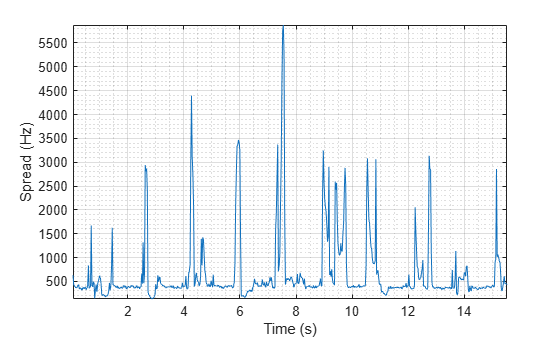Create a `dsp.AudioFileReader` object to read in audio data frame-by-frame. Create a `dsp.SignalSink` to log the spectral spread calculation.

```fileReader = dsp.AudioFileReader('Counting-16-44p1-mono-15secs.wav'); logger = dsp.SignalSink;```

In an audio stream loop:

1. Read in a frame of audio data.

2. Calculate the spectral spread for the frame of audio.

3. Log the spectral spread for later plotting.

To calculate the spectral spread for only a given input frame, specify a window with the same number of samples as the input, and set the overlap length to zero. Plot the logged data.

```win = hamming(fileReader.SamplesPerFrame); while ~isDone(fileReader) audioIn = fileReader(); spread = spectralSpread(audioIn,fileReader.SampleRate, ... 'Window',win, ... 'OverlapLength',0); logger(spread) end plot(logger.Buffer) ylabel('Spread (Hz)')```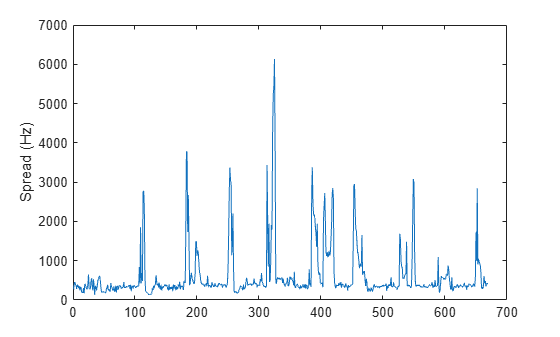Use `dsp.AsyncBuffer` if

• The input to your audio stream loop has a variable samples-per-frame.

• The input to your audio stream loop has an inconsistent samples-per-frame with the analysis window of `spectralSpread`.

• You want to calculate the spectral spread for overlapped data.

Create a `dsp.AsyncBuffer` object, reset the logger, and release the file reader.

```buff = dsp.AsyncBuffer; reset(logger) release(fileReader)```

Specify that the spectral spread is calculated for 50 ms frames with a 25 ms overlap.

```fs = fileReader.SampleRate; samplesPerFrame = round(fs*0.05); samplesOverlap = round(fs*0.025); samplesPerHop = samplesPerFrame - samplesOverlap; win = hamming(samplesPerFrame); while ~isDone(fileReader) audioIn = fileReader(); write(buff,audioIn); while buff.NumUnreadSamples >= samplesPerHop audioBuffered = read(buff,samplesPerFrame,samplesOverlap); spread = spectralSpread(audioBuffered,fs, ... 'Window',win, ... 'OverlapLength',0); logger(spread) end end release(fileReader)```

Plot the logged data.

```plot(logger.Buffer) ylabel('Spread (Hz)')```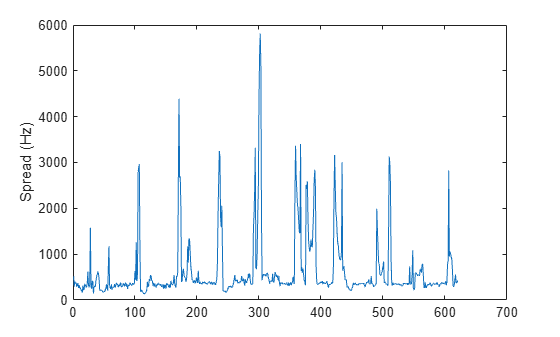## Input Arguments

collapse all

Input signal, specified as a vector, matrix, or 3-D array. How the function interprets `x` depends on the shape of `f`.

Data Types: `single` | `double`

Sample rate or frequency vector in Hz, specified as a scalar or vector, respectively. How the function interprets `x` depends on the shape of `f`:

• If `f` is a scalar, `x` is interpreted as a time-domain signal, and `f` is interpreted as the sample rate. In this case, `x` must be a real vector or matrix. If `x` is specified as a matrix, the columns are interpreted as individual channels.

• If `f` is a vector, `x` is interpreted as a frequency-domain signal, and `f` is interpreted as the frequencies, in Hz, corresponding to the rows of `x`. In this case, `x` must be a real L-by-M-by-N array, where L is the number of spectral values at given frequencies of `f`, M is the number of individual spectra, and N is the number of channels.

• The number of rows of `x`, L, must be equal to the number of elements of `f`.

Data Types: `single` | `double`

### Name-Value Arguments

Specify optional pairs of arguments as `Name1=Value1,...,NameN=ValueN`, where `Name` is the argument name and `Value` is the corresponding value. Name-value arguments must appear after other arguments, but the order of the pairs does not matter.

Before R2021a, use commas to separate each name and value, and enclose `Name` in quotes.

Example: `Window=hamming(256)`

Note

The following name-value arguments apply if `x` is a time-domain signal. If `x` is a frequency-domain signal, name-value arguments are ignored.

Window applied in the time domain, specified as a real vector. The number of elements in the vector must be in the range [1, `size(x,1)`]. The number of elements in the vector must also be greater than `OverlapLength`.

Data Types: `single` | `double`

Number of samples overlapped between adjacent windows, specified as an integer in the range [0, `size(Window,1)`).

Data Types: `single` | `double`

Number of bins used to calculate the DFT of windowed input samples, specified as a positive scalar integer. If unspecified, `FFTLength` defaults to the number of elements in the `Window`.

Data Types: `single` | `double`

Frequency range in Hz, specified as a two-element row vector of increasing real values in the range [0, `f`/2].

Data Types: `single` | `double`

Spectrum type, specified as `"power"` or `"magnitude"`:

• `"power"` –– The spectral spread is calculated for the one-sided power spectrum.

• `"magnitude"` –– The spectral spread is calculated for the one-sided magnitude spectrum.

Data Types: `char` | `string`

## Output Arguments

collapse all

Spectral spread in Hz, returned as a scalar, vector, or matrix. Each row of `centroid` corresponds to the spectral spread of a window of `x`. Each column of `spread` corresponds to an independent channel.

Spectral centroid in Hz, returned as a scalar, vector, or matrix. Each row of `centroid` corresponds to the spectral centroid of a window of `x`. Each column of `centroid` corresponds to an independent channel.

## Algorithms

The spectral spread is calculated as described in :

`$\text{spread}=\sqrt{\frac{\sum _{k={b}_{1}}^{{b}_{2}}{\left({f}_{k}-{\mu }_{1}\right)}^{2}{s}_{k}}{\sum _{k={b}_{1}}^{{b}_{2}}{s}_{k}}}$`

where

• fk is the frequency in Hz corresponding to bin k.

• sk is the spectral value at bin k.

• b1 and b2 are the band edges, in bins, over which to calculate the spectral spread.

• μ1 is the spectral centroid, calculated as described by the `spectralCentroid` function.

 Peeters, G. "A Large Set of Audio Features for Sound Description (Similarity and Classification) in the CUIDADO Project." Technical Report; IRCAM: Paris, France, 2004.